The Japanese Bonsai specialist
Direct order Contact Help / Services NewsletterRhodohypoxis 'pink shot' 1.4 litre pot

Rhodohypoxis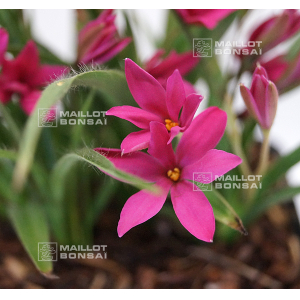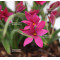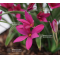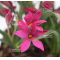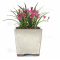ref. : 11183

6,40

Available quantity : 2Order

Description

Vivacious plant, rhizomes. Leaves 4/5 cm. Delivered as a strong two-year-old tuft in a plastic 12*12 cm pot.

At the start of summer the rhodohypoxis 'pink shot' spiky green leaves are surrounded by numerous 1/1.5 cm dark pink flowers. Flowering lasts for between 3 and 4 weeks. The foliage dies back in winter when it's best to keep the plant away from frosts in a cold greenhouse with almost no water.

#pink 4.4 #rhodohypoxis 4.2 #shot 3.4 #kusamono 2.8 #plants 2.6 #leaves 2.6 #litre 2.5 #plant 2.5 #small 2.5 #greenhouse 2

Formule
(( ROUND((CHAR_LENGTH(b.article_nom)-CHAR_LENGTH(REPLACE(b.article_nom, 'pink', '')))/LENGTH('pink')) + ROUND((CHAR_LENGTH(b.article_description)-CHAR_LENGTH(REPLACE(b.article_description, 'pink', '')))/LENGTH('pink')) ) * 4.4) + (( ROUND((CHAR_LENGTH(b.article_nom)-CHAR_LENGTH(REPLACE(b.article_nom, 'rhodohypoxis', '')))/LENGTH('rhodohypoxis')) + ROUND((CHAR_LENGTH(b.article_description)-CHAR_LENGTH(REPLACE(b.article_description, 'rhodohypoxis', '')))/LENGTH('rhodohypoxis')) ) * 4.2) + (( ROUND((CHAR_LENGTH(b.article_nom)-CHAR_LENGTH(REPLACE(b.article_nom, 'shot', '')))/LENGTH('shot')) + ROUND((CHAR_LENGTH(b.article_description)-CHAR_LENGTH(REPLACE(b.article_description, 'shot', '')))/LENGTH('shot')) ) * 3.4) + (( ROUND((CHAR_LENGTH(b.article_nom)-CHAR_LENGTH(REPLACE(b.article_nom, 'leaves', '')))/LENGTH('leaves')) + ROUND((CHAR_LENGTH(b.article_description)-CHAR_LENGTH(REPLACE(b.article_description, 'leaves', '')))/LENGTH('leaves')) ) * 2.6) + (( ROUND((CHAR_LENGTH(b.article_nom)-CHAR_LENGTH(REPLACE(b.article_nom, 'litre', '')))/LENGTH('litre')) + ROUND((CHAR_LENGTH(b.article_description)-CHAR_LENGTH(REPLACE(b.article_description, 'litre', '')))/LENGTH('litre')) ) * 2.5) + (( ROUND((CHAR_LENGTH(b.article_nom)-CHAR_LENGTH(REPLACE(b.article_nom, 'plant', '')))/LENGTH('plant')) + ROUND((CHAR_LENGTH(b.article_description)-CHAR_LENGTH(REPLACE(b.article_description, 'plant', '')))/LENGTH('plant')) ) * 2.5) + (( ROUND((CHAR_LENGTH(b.article_nom)-CHAR_LENGTH(REPLACE(b.article_nom, 'surrounded', '')))/LENGTH('surrounded')) + ROUND((CHAR_LENGTH(b.article_description)-CHAR_LENGTH(REPLACE(b.article_description, 'surrounded', '')))/LENGTH('surrounded')) ) * 2) + (( ROUND((CHAR_LENGTH(b.article_nom)-CHAR_LENGTH(REPLACE(b.article_nom, 'greenhouse', '')))/LENGTH('greenhouse')) + ROUND((CHAR_LENGTH(b.article_description)-CHAR_LENGTH(REPLACE(b.article_description, 'greenhouse', '')))/LENGTH('greenhouse')) ) * 2) + (( ROUND((CHAR_LENGTH(b.article_nom)-CHAR_LENGTH(REPLACE(b.article_nom, 'vivacious', '')))/LENGTH('vivacious')) + ROUND((CHAR_LENGTH(b.article_description)-CHAR_LENGTH(REPLACE(b.article_description, 'vivacious', '')))/LENGTH('vivacious')) ) * 1.9) + (( ROUND((CHAR_LENGTH(b.article_nom)-CHAR_LENGTH(REPLACE(b.article_nom, 'delivered', '')))/LENGTH('delivered')) + ROUND((CHAR_LENGTH(b.article_description)-CHAR_LENGTH(REPLACE(b.article_description, 'delivered', '')))/LENGTH('delivered')) ) * 1.9)

Secure paymentDelivery

Our logistic partners :04 74 55 23 48
Pépinière MAILLOT-BONSAÏ
Le Bois Frazy
01990 RELEVANT - FRANCE
on appointment